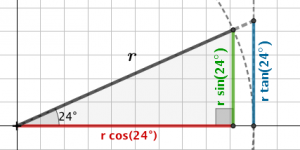# SOH CAH TOA Finding a side

Online Instruction from Mathisfun and Khan Academy

We have already that the sine of an angle can be defined as the side opposite the angle on a right angle triangle that has hypotenuse length 1. The cosine is the length of the adjacent side, and the tangent is the length on the tangent as seen in the diagram:For every other right angled triangle, we see that the scale factor is just the length of the hypotenuse:Now let’s focus on the side opposite the marked angle, the green side. We see thator, in general,Which leads us to the formula:Similarly, the adjacent side, the red length can be calculated as:Finally, through examining the triangle made with the tangent line:We see that in this case, the side opposite the angle divided by the side adjacent gives us:This leads us to three tidy trig formulae for right angled triangles:Which leads us to the mnemonic: SOH CAH TOA, used for calculating sides and angles on any right angled triangle.

# Finding a side on a right angled triangle

Use the sine; cosine or tangent ratio to find the side indicated.

Draw the triangle onto paper. Label, and show working clearly.

Example SOH CAH TOA